Sunday, January 6, 2008

Irodov Problem 1.114Basically, the body will experience two kinds of inertial forces when seen from the rotating frame of the disc, i) the centripetal force and ii) the Coriolis force.
What I shall do is derive everything from first principles so that the origin of these inertial forces is clear starting from as seen from the point of view of a stationary observer.

Suppose that at some instant the center of the disc is at an angle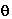and the particle is aligned at an anglewith the diameter passing through the axis. The position vector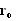of the particle with respect to a stationary observer located at the intersection point of the disc and the axis of rotation is then given by,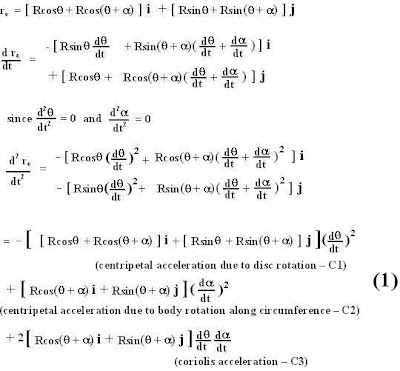Since both the disc's rotation about the axis and the particle's rotation about the center of the disc in the disc frame are constant the second derivatives ofandare 0. As seen in the derivation and the second figure, the body experiences three kinds of accelerations, i) the centripetal acceleration due to the discs rotation direction towards the axis of rotation - C1, ii) the centripetal acceleration of the body due to its rotation along the circumference of the disc directed towards the disc's center - C2 and iii) the Coriolis acceleration directed towards the center of the disc - C3.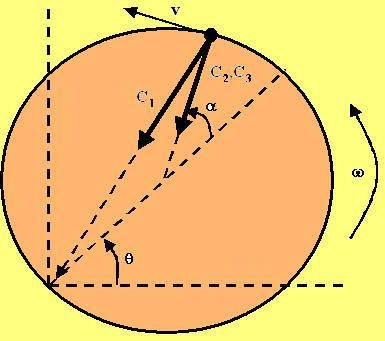Now let us consider an observer fixed to the rotating frame of the disc. With respect to this rotating observer, the body is simply rotating around the disc's circumference at a constant rate
at a rate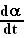. The acceleration of the body as seen by this observer is thus simplydirected towards the center of the disc (C2). Thus, the remaining components of acceleration (C1 and C3) will be seen as inertial forces in this rotating frame of the disc (acting the opposite direction to the directions of the accelerations).

The problem says, the body moves in such a manner that the sum total of the inertial forces when the body is at the farthest point (i.e diametrically opposite to the axis or when=0) in this frame is 0. In other words, when=0, C1 + C3=0. Substituting this condition in the above derivation in (1) we have,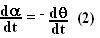In other words the body should rotate along the circumference of the disc at the same angular velocity as the disc () but in the opposite direction. This will ensure that the Coriolis force opposes the centripetal force and exactly cancel it when the body is at the farthest point from the axis.

a) To an observer of the disc thus the acceleration of the body will be C2 =.

b) The inertial forces as seen by the observer will thus be the sum of C1 (centripetal force acting outwards) and C3 (Coriolis force acting towards the center of the disc) as shown in the figure below.Let the particle be a distance r from the axis (AD=r) . Let CB be the perpendicular on AD, then AB=BD=r/2. Also angle BAC =. Thus, we have,Anonymous said...

Sir why have u stopped?

It is some excellent work and I appreciate it to the core of my heart.....
Plz don'tpause.
I been a regular visitor plz don't let me down...
I'm giving the JEE this yr

Krishna Kant Chintalapudi said...

thank you very much for your appreciation. I was just a bit busy with work. I will get back to posting the solutions...